Test Description

## 10 Questions MCQ Test International Mathematics Olympiad (IMO) for Class 10 | Math Olympiad Test: Quadratic Equations- 4

 1 Crore+ students have signed up on EduRev. Have you?

### If the roots of the equation (a2 + b2) x2 – 2b(a + c)x + (b2 + c2) = 0 are equal, then ________.

Detailed Solution for Math Olympiad Test: Quadratic Equations- 4 - Question 1

Since roots of the given equation are equal
∴ D = 0
⇒ (–2b(a + c))2 – 4(a2 + b2)(b2 + c2) = 0
⇒ 4b2(a2 + c2 + 2ac) – 4(a2b2 + a2c2 + b4 + b2c2) = 0
⇒ a2b2 + b2c2 + 2ab2c – a2b2 – a2c2 – b4 – b2c2 = 0
⇒ 2ab2c – a2c2 – b4 = 0 ⇒ b4 + a2c2 – 2ab2c = 0
⇒ (b2 – ac)2 = 0 ⇒ b2 = ac

### The roots of the equation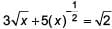can be found by solving ________.

Detailed Solution for Math Olympiad Test: Quadratic Equations- 4 - Question 2

We have, 3x1/2 + 5x−1/2 = √2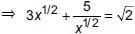⇒ 3x + 5 = (2x)1/2 ...(1)
Squaring both sides of (1), we have
9x2 + 25 + 30x = 2x
⇒ 9x2 + 28x + 25 = 0

### The roots of the equation x2/3 + x1/3 – 2 = 0 are ________.

Detailed Solution for Math Olympiad Test: Quadratic Equations- 4 - Question 3

Given equation is x2/3 + x1/3 – 2 = 0
Let y = x1/3 ⇒ y2 + y – 2 = 0
⇒ y2 + 2y – y – 2 = 0 ⇒ (y – 1)(y + 2) = 0
⇒ y = 1 or y = –2 ⇒ x1/3 = 1 or x1/3 = –2
⇒ x = 1 or x = – 8

Two numbers whose sum is 12 and the absolute value of whose difference is 4 are the roots of the equation ________.

Detailed Solution for Math Olympiad Test: Quadratic Equations- 4 - Question 4

Let the two roots be a and b, then a + b = 12 ...(1) and a – b = 4 ...(2)
⇒ a = 8 and b = 4 (from (1) and (2))
∴ Required equation is x2 – 12x + 32 = 0

In a bangle shop, if the shopkeeper displays the bangles in the form of a square then he is left with 38 bangles. If he wanted to increase the size of square by one unit each side of the square he found that 25 bangles fall short of in completing the square. The actual number of bangles which he had with him in the shop was ________.

Detailed Solution for Math Olympiad Test: Quadratic Equations- 4 - Question 5

Let the number of bangles in a side of square = x
According to the question, x2 + 38 = Total no. of bangles ...(1)
Also, (x + 1)2 – 25 = Total no. of bangles ...(2)
From (1) and (2), we have
x2 + 38 = (x + 1)2 – 25
⇒ 38 + 24 = 2x ⇒ x = 31
∴ Total no. of bangles = (31)2 + 38 = 999

In the equation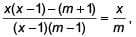the roots are equal when m = ________.

Detailed Solution for Math Olympiad Test: Quadratic Equations- 4 - Question 6

We have,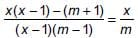⇒ (x2 – x)m – (m2 + m) = x(x – 1)(m – 1)
⇒ x2m – mx – m2 – m = x2m – x2 – xm + x
⇒ (x2 – m2) – (m + x) = 0
⇒ (x + m)(x – m – 1) = 0
Now, since roots are equal ⇒ –m = m + 1 ⇒ m = -(1/2)

In the Maths Olympiad of 2020 at Animal Planet, two representatives from the donkey’s side, while solving a quadratic equation, committed the following mistakes.
(i) One of them made a mistake in the constant term and got the roots as 5 and 9.
(ii) Another one committed an error in the coefficient of x and he got the roots as 12 and 4.
But in the meantime, they realised that they are wrong and they managed to get it right jointly. Find the quadratic equation.

Detailed Solution for Math Olympiad Test: Quadratic Equations- 4 - Question 7

For 1st one,
Let the equation be x2 + ax + b = 0
Since roots are 5 and 9
∴ a = –14 and b = 45
For 2nd one,
Let the equation be x2 + px + q = 0
Since roots are 12 and 4.
∴ p = –16 and q = 48
Now, according to the question, b and p both are wrong. Therefore, the correct equation would be x2 –14x + 48 = 0

A man walks a distance of 48 km in a given time. If he walks 2 km/hr faster, he will perform the journey 4 hrs before. His normal rate of walking, is ________.

Detailed Solution for Math Olympiad Test: Quadratic Equations- 4 - Question 8

Let the speed of man be x km/hr and the time taken by him to cover 48 km with speed x be t
According to the question, 48 = x × t ...(1)
Also, 48 = (x + 2)(t – 4) ...(2)
⇒ 48 = xt – 4x + 2t – 8
⇒ 56 = 48 – 4x + 2t [Using (1)]
⇒ 8 = – 4x + 2t ⇒ 4 = –2x + t
⇒ 4 = −2x + (48/x) [Using (1)]
⇒ 2x = –x2 + 24 ⇒ x2 + 2x – 24 = 0
⇒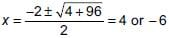Since, speed cannot be negative
∴ Required speed is 4 km/hr.

Swati can row her boat at a speed of 5 km/hr in still water. If it takes her 1 hour more to row the boat 5.25 km upstream than to return downstream, find the speed of the stream.

Detailed Solution for Math Olympiad Test: Quadratic Equations- 4 - Question 9

Let the speed of the stream be x km/h
Speed of the boat in upstream = (5 - x)km/h
Speed of the boat in downstream = (5 + x)km/h
Time, say t1 (in hours), for going 5.25 km upstream = 5.25/5 - x
Time, say t2 (in hours), for returning 5.25 km downstream = 5.25/5 + x
Obviously t1 > t2
Therefore, according to the given condition of the problem,
t1 = t2 + 1This gives x = 2, since we reject x = -25/2
Thus, the speed of the stream is 2 km/h.

₹ 6500 were divided equally among a certain number of persons. If there had been 15 more persons, each would have got ₹ 30 less. Find the original number of persons.

Detailed Solution for Math Olympiad Test: Quadratic Equations- 4 - Question 10

Let x be the number of persons and y be the amount received by each person.
According to the question, xy = 6500 ...(1)
Also, (x + 15)(y – 30) = 6500 ...(2)
⇒ xy – 30x + 15y – 450 = 6500
⇒ 6500 – 30x + 15y – 450 = 6500 [From (1)]
⇒ 2x – y + 30 = 0
⇒ 2x - (6500/x) + 30 = 0 [From (1)]
⇒ 2x2 – 6500 + 30x = 0
⇒ x2 + 15x – 3250 = 0
⇒ (x – 50)(x + 65) = 0
⇒ x = 50 or x = – 65
Since, number of persons cannot be negative. Hence, number of persons = 50

## International Mathematics Olympiad (IMO) for Class 10

22 docs|62 tests
 Use Code STAYHOME200 and get INR 200 additional OFF Use Coupon Code